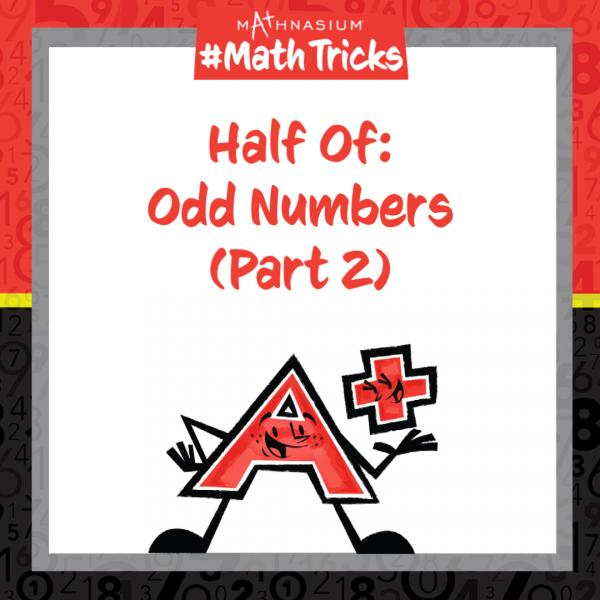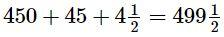* indicates a required field• phone number format invalid• email format invalidProblems detected, please review the form.
Privacy - Terms

We are OPEN for in-centre AND online instruction. Please call or email us for further information.

Feb 17, 2022

#Welcome to Mathnasium’s Math Tricks series. Our previous Math Tricks have looked at strategies for finding half of odd numbers whose tens digits are even. Today we are focusing on a strategy to find the half of any odd number, such as 99… or 999.

Calculating half of anything means to find “two parts the same,” “halfway between,” or, finding “what number plus itself is equal to that number.” Follow along with the example below as we find half of 999.

Step 1: Decompose the whole into its parts.

999 is nine hundreds, nine tens, and nine ones.

Step 2: Find half of the whole by finding half of each part.

Half of 999 = Half of 900 + Half of 90 + Half of 9.

Step 3: Calculate the half of each part.

Half of 9 is four-and-a-half.

Half of 90 is 45 (not forty-and-a-half).

Half of 900 (or nine hundreds), is four-and-a-half hundreds, or 450.

Step 4: Find the half of the whole by summing up the half of each part.

Half of 999 is equal to450+45+412=49912.

We can check that.

We have calculated half of nine hundred ninety-nine to be four hundred ninety-nine-and-a-half. Now, with this strategy, you are ready to find half of any odd number. Click here for more practice problems!

If you missed our Math Tricks video on the Half of Odd Numbers (Part 2), check it out on Facebook page:  https://fb.watch/beedKOdd54/